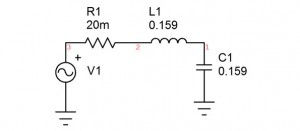top of page
Search

# Understanding Voltage Response Of An LC CircuitFor an undamped LC circuit excited by an AC voltage source through simple phaser equations, we come to see that the circuit becomes short circuited at the resonant frequency. However if we analyze the voltage gain across the capacitor we also see that the gain becomes infinite at the resonant frequency. I have doubts.

On one end we are saying that the voltage gain is infinite and also saying that the circuit is short circuited. Can we prove that the voltage on the capacitor accumulates to infinite voltage over a period of time? (Because it just seems unlikely that the voltage built up across the capacitor will suddenly be infinite within the first ac cycle of the supply voltage being turned on. I am assuming that the source is a sine voltage and at t=0,the voltage is also.

A simple LC circuit is shown in Fig. 1.  The circuit is underdamped and the Q is controlled by R1, which can be in series with L1, in series with C2 or proportioned between the two.  For simplicity, the circuit is normalized to a 1 Hz resonant frequency and a characteristic impedance of 1 ohm, defined by the equal values of L1 and C1.Figure 1

At resonance, the input resistance is 1/R1, so the Q is 3 as shown.  At resonance the impedance of the capacitor is 1 ohm.  Therefore, placing a 1 V peak sine wave at the resonant frequency results in 3 Apk and the voltage across the capacitor is 3 V pk.  At a Q of 10 the resistance is 0.1 ohm and a 1 Vpk sine wave at the resonant frequency results in 3 A peak and the voltage across the capacitor is 3 V peak.  Increasing the Q further to 50, the resistor is 20 milliohm and the current for a 1 V peak sine wave is 50 A peak resulting in 50 V across the capacitor.  If the Q is infinite then the resistance is 0 and the current is infinite.  The voltage across the capacitor is then infinity times the characteristic impedance (1 ohm) or infinity.